# Limit is linear

## Statement

### In terms of additivity and pulling out scalars

Suppose$f$ and$g$ are functions of one variable. Suppose$c \in \R$ is such that both$f$ and$g$ are defined on the immediate left and the immediate right of$c$. Further, suppose that the limits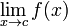$\lim_{x \to c} f(x)$ and$\lim_{x \to c} g(x)$ both exist (as finite numbers). In that case, the limit of the pointwise sum of functions$f + g$ exists and is the sum of the individual limits: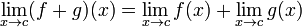$\lim_{x \to c} (f + g)(x) = \lim_{x \to c} f(x) + \lim_{x \to c} g(x)$

An equivalent formulation:$\! \lim_{x \to c} [f(x) + g(x)] = \lim_{x \to c} f(x) + \lim_{x \to c} g(x)$

Scalars: Suppose$f$ is a function of one variable and$\lambda$ is a real number. Suppose$c \in \R$ is such that$f$ is defined on the immediate left and immediate right of$c$, and that$\lim_{x \to c} f(x)$ exists. Then: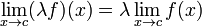$\lim_{x \to c} (\lambda f)(x) = \lambda \lim_{x \to c} f(x)$

An equivalent formulation:$\lim_{x \to c} \lambda f(x) = \lambda \lim_{x \to c} f(x)$

### In terms of generalized linearity

Suppose$f_1,f_2,\dots,f_n$ are functions and$a_1,a_2,\dots,a_n$ are real numbers.$\lim_{x \to c} [a_1f_1(x) + a_2f_2(x) + \dots + a_nf_n(x)] = a_1\lim_{x \to c} f_1(x) + a_2\lim_{x \to c}f_2(x) + \dots + a_n\lim_{x \to c} f_n(x)$

if the right side expression makes sense.

In particular, setting$n = 2, a_1 = 1, a_2 = -1$, we get that the limit of the difference is the difference of the limits.

### One-sided version

One-sided limits (i.e., the left hand limit and the right hand limit) are also linear. In other words, we have the following, whenever the respective right side expressions make sense:

•$\! \lim_{x \to c^-} [f(x) + g(x)] = \lim_{x \to c^-} f(x) + \lim_{x \to c^-} g(x)$
•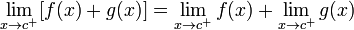$\! \lim_{x \to c^+} [f(x) + g(x)] = \lim_{x \to c^+} f(x) + \lim_{x \to c^+} g(x)$
•$\! \lim_{x \to c^-} \lambda f(x) = \lambda \lim_{x \to c^-} f(x)$
•$\! \lim_{x \to c^+} \lambda f(x) = \lambda \lim_{x \to c^+} f(x)$
•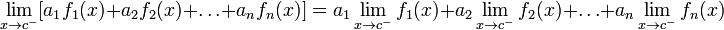$\lim_{x \to c^-} [a_1f_1(x) + a_2f_2(x) + \dots + a_nf_n(x)] = a_1\lim_{x \to c^-} f_1(x) + a_2\lim_{x \to c^-}f_2(x) + \dots + a_n\lim_{x \to c^-} f_n(x)$
•$\lim_{x \to c^+} [a_1f_1(x) + a_2f_2(x) + \dots + a_nf_n(x)] = a_1\lim_{x \to c^+} f_1(x) + a_2\lim_{x \to c^+}f_2(x) + \dots + a_n\lim_{x \to c^+} f_n(x)$